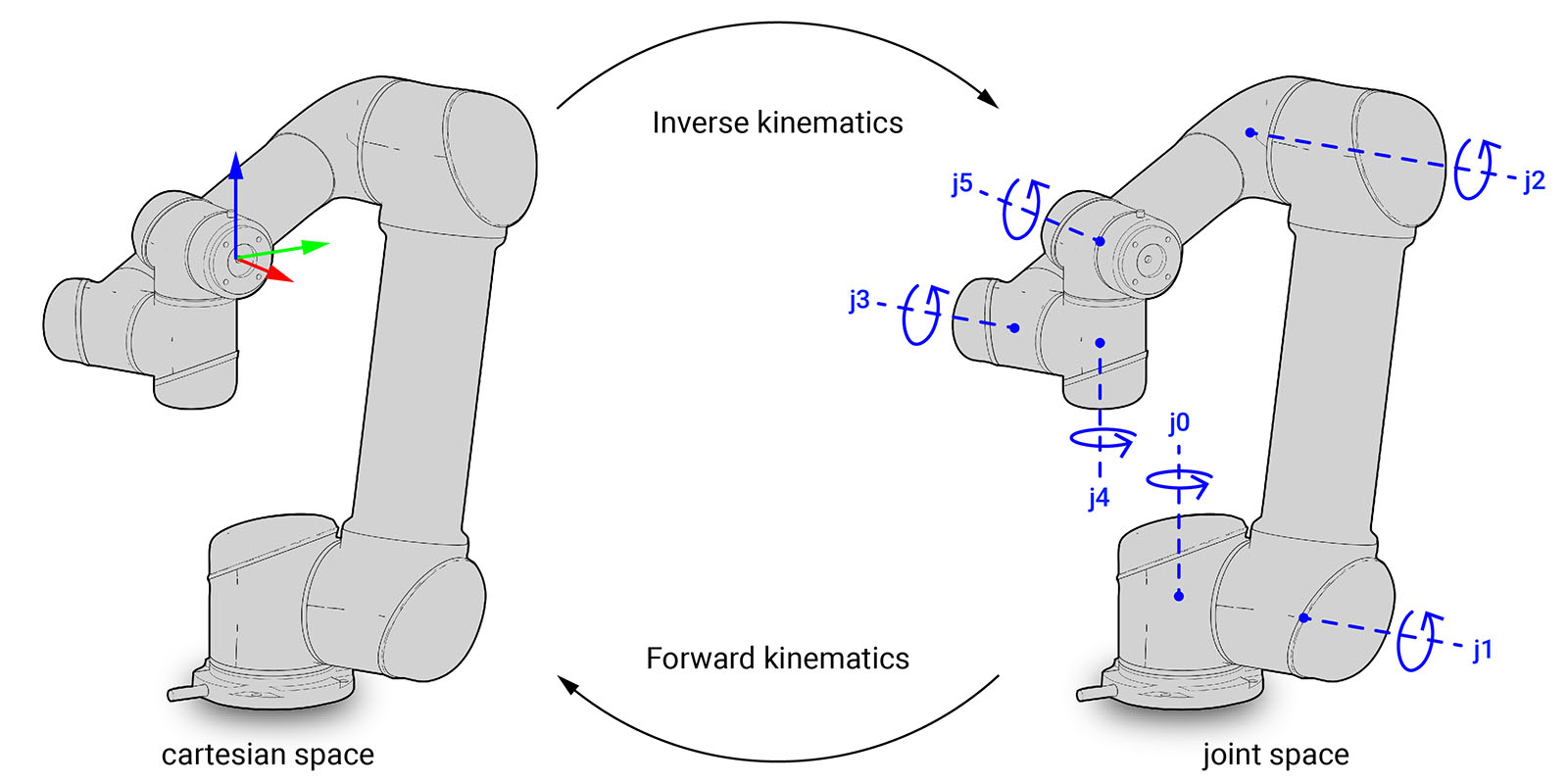# 3.2. Forward and inverse kinematicsNote

The following examples use the ROS backend and the MoveI! planner for UR5 robots. Before running them, please make sure you have the ROS backend correctly configured and the UR5 Planner started.

## 3.2.1. Forward kinematics

The forward kinematics function calculates the pose of the robot’s end-effector from joint states (cartesian space to joint space). This means the state of each joint in the articulated body of a robot needs to be defined.

Joint states are described in COMPAS FAB with the compas_fab.robots.Configuration class.

The simplest way to calculate forward kinematics is based on the properties defined by the robot model and does not require ROS to be running:

from compas_fab.robots import Configuration
from compas_fab.robots.ur5 import Robot

robot = Robot()
configuration = Configuration.from_revolute_values([-2.238, -1.153, -2.174, 0.185, 0.667, 0.])

frame_WCF = robot.forward_kinematics(configuration)

print("Frame in the world coordinate system")
print(frame_WCF)

assert str(frame_WCF.point) == 'Point(0.300, 0.100, 0.500)'


Additionally, if the compas_fab.robots.Robot is assigned a client, it will try to use it to resolve forward kinematics. The following example shows the same solutions but calculated by ROS:

from compas_fab.backends import RosClient
from compas_fab.robots import Configuration
from compas_fab.robots.ur5 import Robot

with RosClient() as client:
robot = Robot(client)
configuration = Configuration.from_revolute_values([-2.238, -1.153, -2.174, 0.185, 0.667, 0.])

frame_WCF = robot.forward_kinematics(configuration)

print("Frame in the world coordinate system")
print(frame_WCF)


## 3.2.2. Inverse kinematics

Inverse kinematics is the inverse function of forward kinematics. The inverse kinematics function calculates the joint states required for the end-effector to reach a certain target pose (joint space to cartesian space).

The following code exemplifies how to calculate this:

from compas.geometry import Frame
from compas_fab.backends import RosClient
from compas_fab.robots.ur5 import Robot

with RosClient() as client:
robot = Robot(client)

frame_WCF = Frame([0.3, 0.1, 0.5], [1, 0, 0], [0, 1, 0])
start_configuration = robot.zero_configuration()

configuration = robot.inverse_kinematics(frame_WCF, start_configuration)

print("Found configuration", configuration)